Question

# In an isothermal and reversible process, 945 J of heat is removed from a system and...

In an isothermal and reversible process, 945 J of heat is removed from a system and transferred to the surroundings. The temperature is 314 K. What is the change in entropy of the system? Justify your answer.

A) –3.01 J/K

B) +3.01 J/K

C) –0.332 J/K

D) +0.332 J/K

E) +2.97 × 105 J/K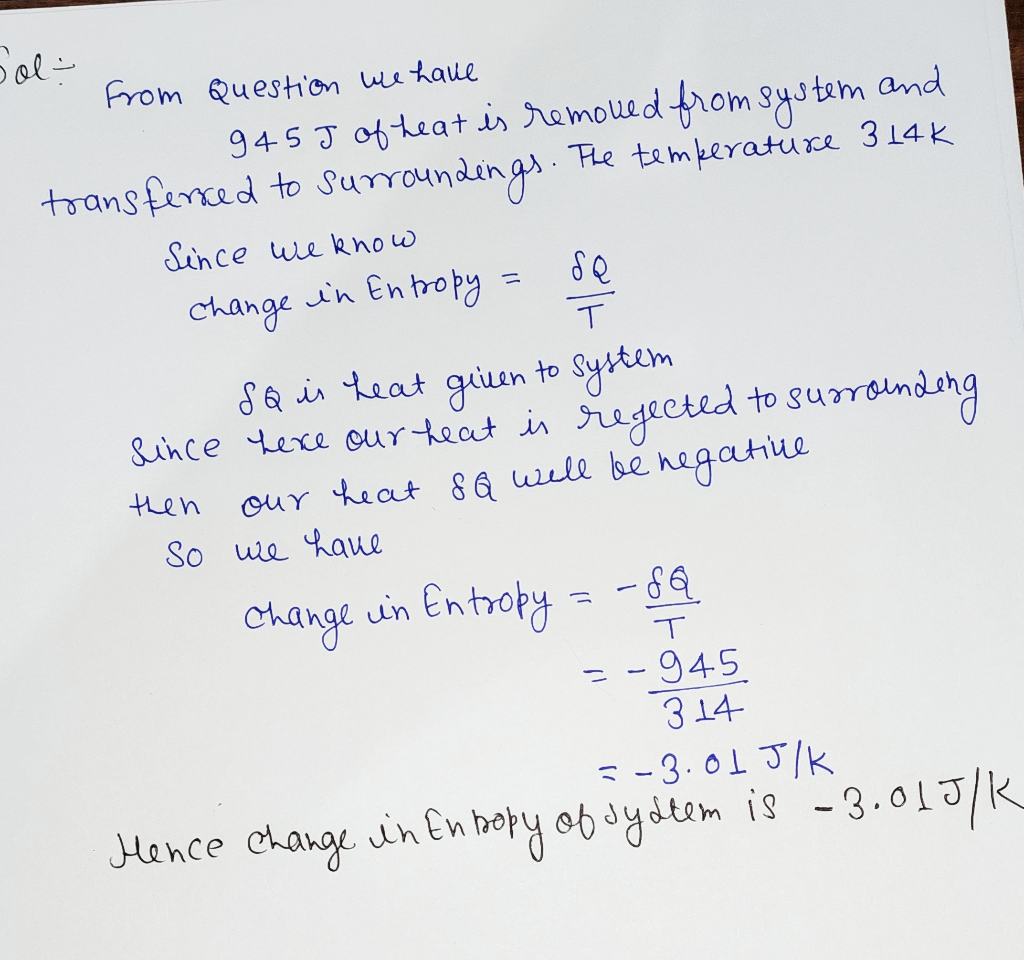#### Earn Coins

Coins can be redeemed for fabulous gifts.

Similar Homework Help Questions
• ### 5. (15) A closed system undergoes a process where heat transfer is allowed along the system...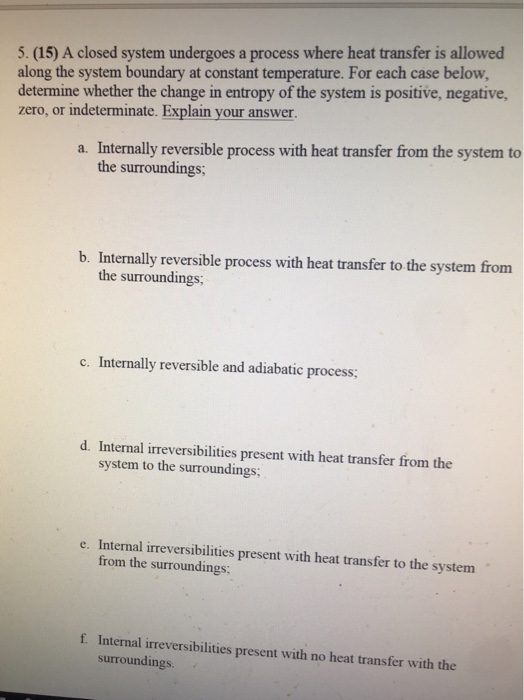5. (15) A closed system undergoes a process where heat transfer is allowed along the system boundary at constant temperature. For each case below, determine whether the change in entropy of the system is positive, negative, zero, or indeterminate. Explain your answer. a. Internally reversible process with heat transfer from the system to the surroundings b. Internally reversible process with heat transfer to the system from the surroundings; Internally reversible and adiabatic process; c. d. Internal irreversibilities present with heat...

• ### (5 pts) 15.A quantity of 2.0 moles of an ideal gas undergoes a reversible isothermal process...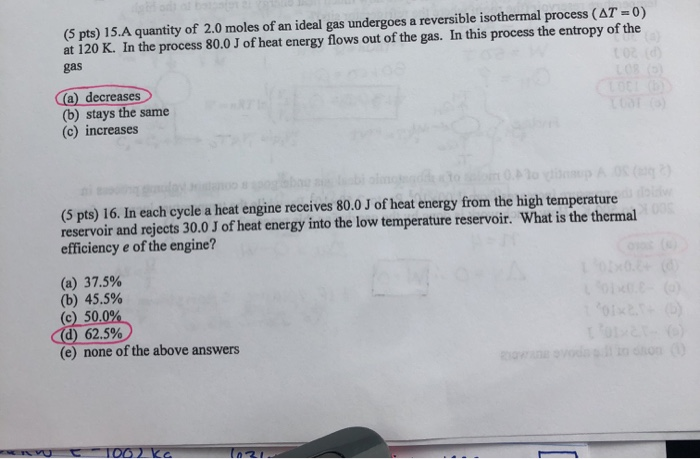(5 pts) 15.A quantity of 2.0 moles of an ideal gas undergoes a reversible isothermal process (AT -0) at 120 K. In the process 80.0 J of heat energy flows out of the gas. In this process the entropy of the gas a decreases (b) stays the same (c) increases (5 pts) 16. In each cycle a heat engine receives 80.0 J of heat energy from the high temperature reservoir and rejects 30.0 J of heat energy into the low...

• ### The combustion process inside a jet engine creates 300 J of heat and does 120 J...

The combustion process inside a jet engine creates 300 J of heat and does 120 J of work spinning a turbine shaft. What is the change in specific enthalpy of the gas? Through an irreversible process, object A transfers 560 J of heat to object B. The resulting temperature of the system is 241 K. What is the entropy of the system? For an ideal gas, if the pressure remains the same but the volume is doubled, what would you...

• ### A process has been proposed whereby an ideal gas is taken from P=10 bar and T=300...

A process has been proposed whereby an ideal gas is taken from P=10 bar and T=300 K to P=1 bar and T=500K in a closed system. During the process the system performs 1,000 [J] of work and receives 6,430 [J] of heat from the surroundings at a constant temperature of 300 K. The constant pressure heat capacity of the gas cp=30 [J/(mol K)] . Gas constant R=8.314 [J/(mol K)]. (a) (7 pts.) What is the change of molar internal energy...

• ### During an isothermal compression of an ideal gas, 410J of heat must be removed from the...

During an isothermal compression of an ideal gas, 410J of heat must be removed from the gas to maintain constant temperature. Part A How much work is done by the gas during the process? Express your answer with the appropriate units.

• ### (1) A process has been proposed whereby an ideal gas is taken from P=10 bar and...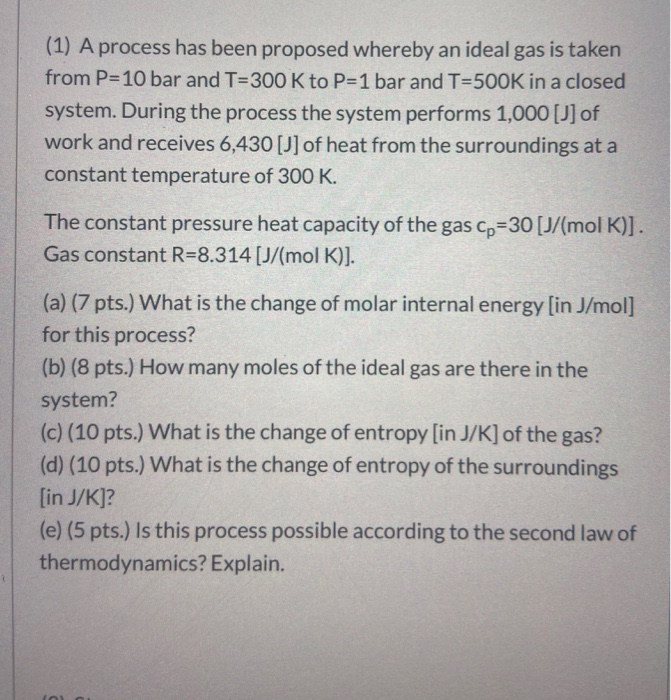(1) A process has been proposed whereby an ideal gas is taken from P=10 bar and T=300 K to P=1 bar and T=500K in a closed system. During the process the system performs 1,000 [J] of work and receives 6,430 [j] of heat from the surroundings at a constant temperature of 300 K. The constant pressure heat capacity of the gas Cp=30 [J/(mol K)]. Gas constant R=8.314 [J/(mol K)]. (a) (7 pts.) What is the change of molar internal energy...

• ### (1) A process has been proposed whereby an ideal gas is taken from P=10 bar and...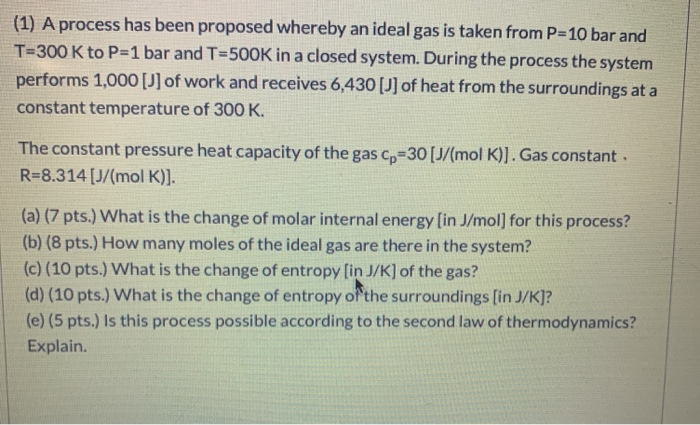(1) A process has been proposed whereby an ideal gas is taken from P=10 bar and T=300 K to P=1 bar and T=500K in a closed system. During the process the system performs 1,000 [j] of work and receives 6,430 [J] of heat from the surroundings at a constant temperature of 300 K. The constant pressure heat capacity of the gas cp=30 [J/mol K)]. Gas constant. R=8.314 [J/(mol K)]. (a) (7 pts.) What is the change of molar internal energy...

• ### 22 g of Co, gas is in a container under 1.50 bar. After isothermal reversible expansion,...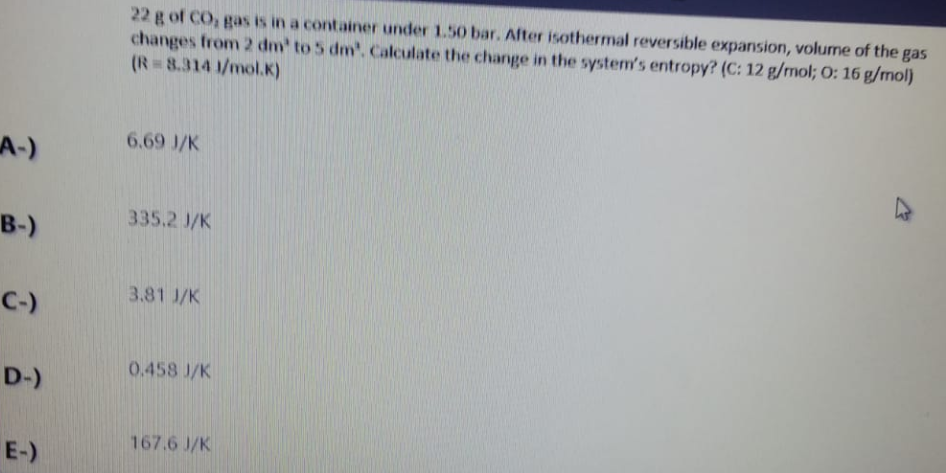22 g of Co, gas is in a container under 1.50 bar. After isothermal reversible expansion, volume of the gas changes from 2 dm to 5 dm'. Calculate the change in the system's entropy? (C: 12 g/mol; O: 16 g/mol) (R-8.314 1/mol.) A-) 6.69 J/K B-) 335.2 J/K C-) 3.81 J/K D-) 0.458 J/K E-) 167.6 J/K

• ### 1. If a system undergoes a reversible process, a it must be possible to restore the...

1. If a system undergoes a reversible process, a it must be possible to restore the system to its original state. b it must be possible to restore the surroundings to their original state. c it must be possible to restore both the system and the surroundings to their original states. d it is impossible to restore either the the system or the surroundings to their original states. e the system must not interact with its surroundings. 2. According to...

• ### During a certain thermodynamic process, 418 J of work are done on a system and 214...

During a certain thermodynamic process, 418 J of work are done on a system and 214 cal of heat are transferred to the system. The change in internal energy during the process is Group of answer choices a. 314 cal. b. 114 cal. c. 468 cal. d. 368 cal. e. 632 cal.